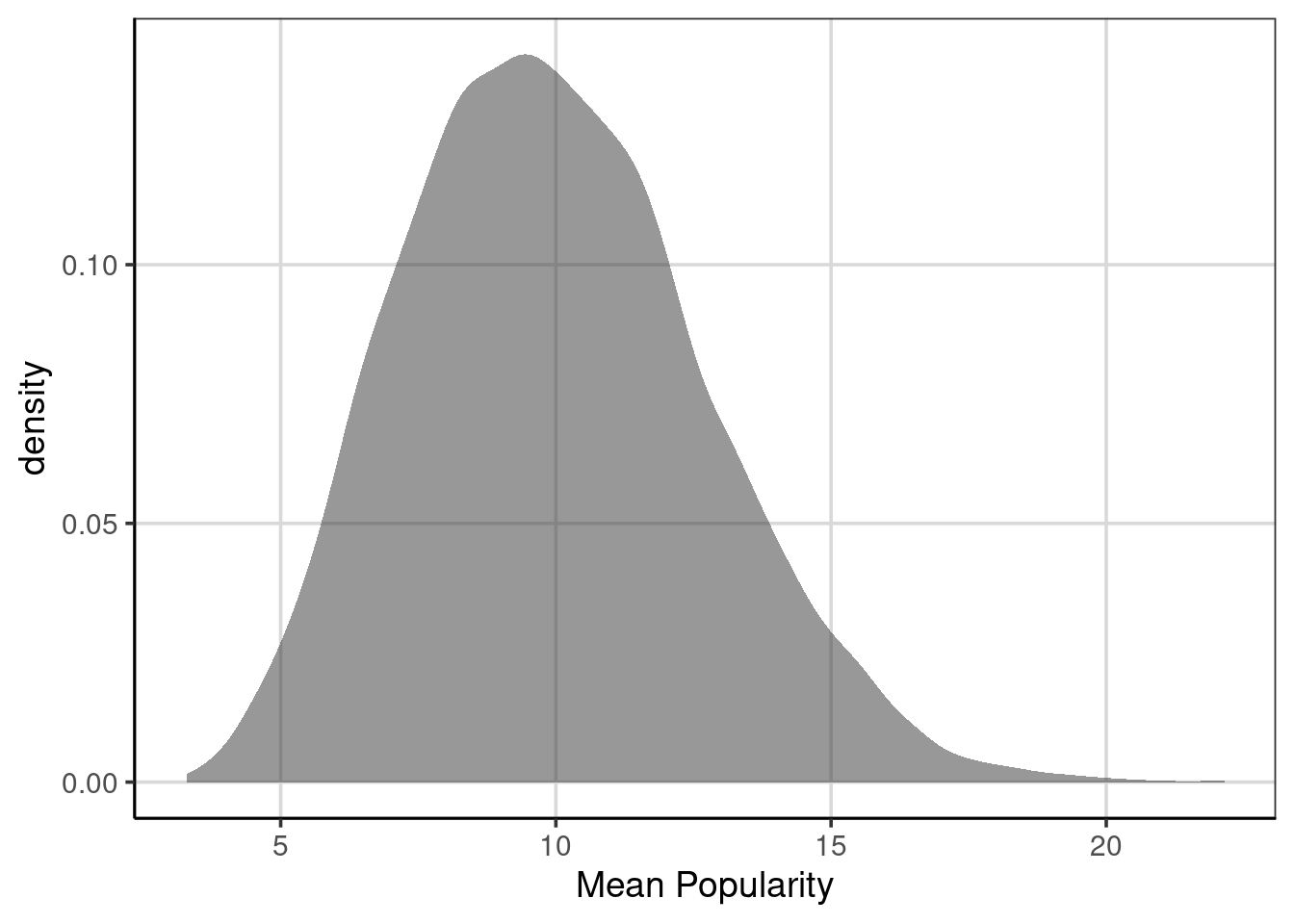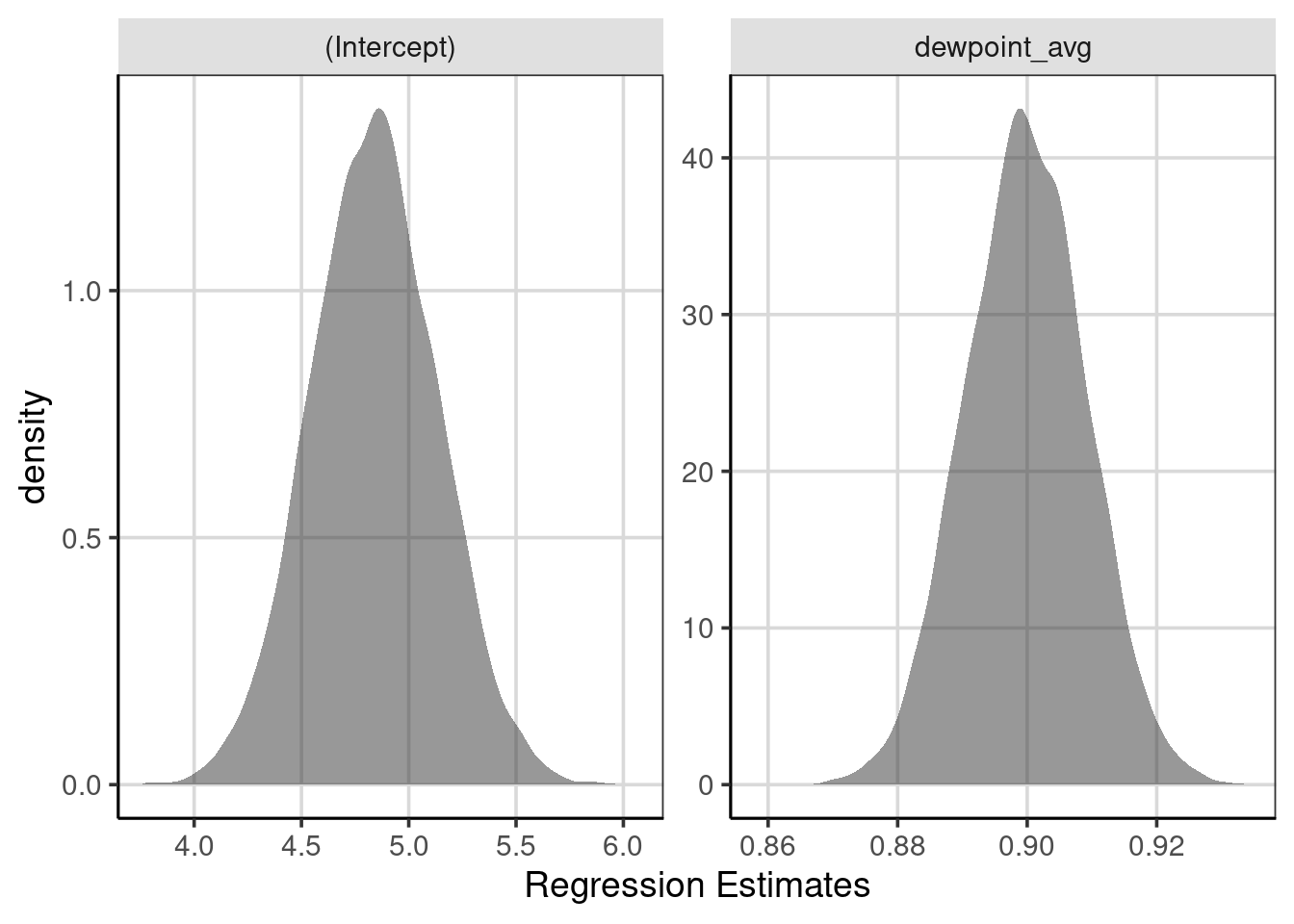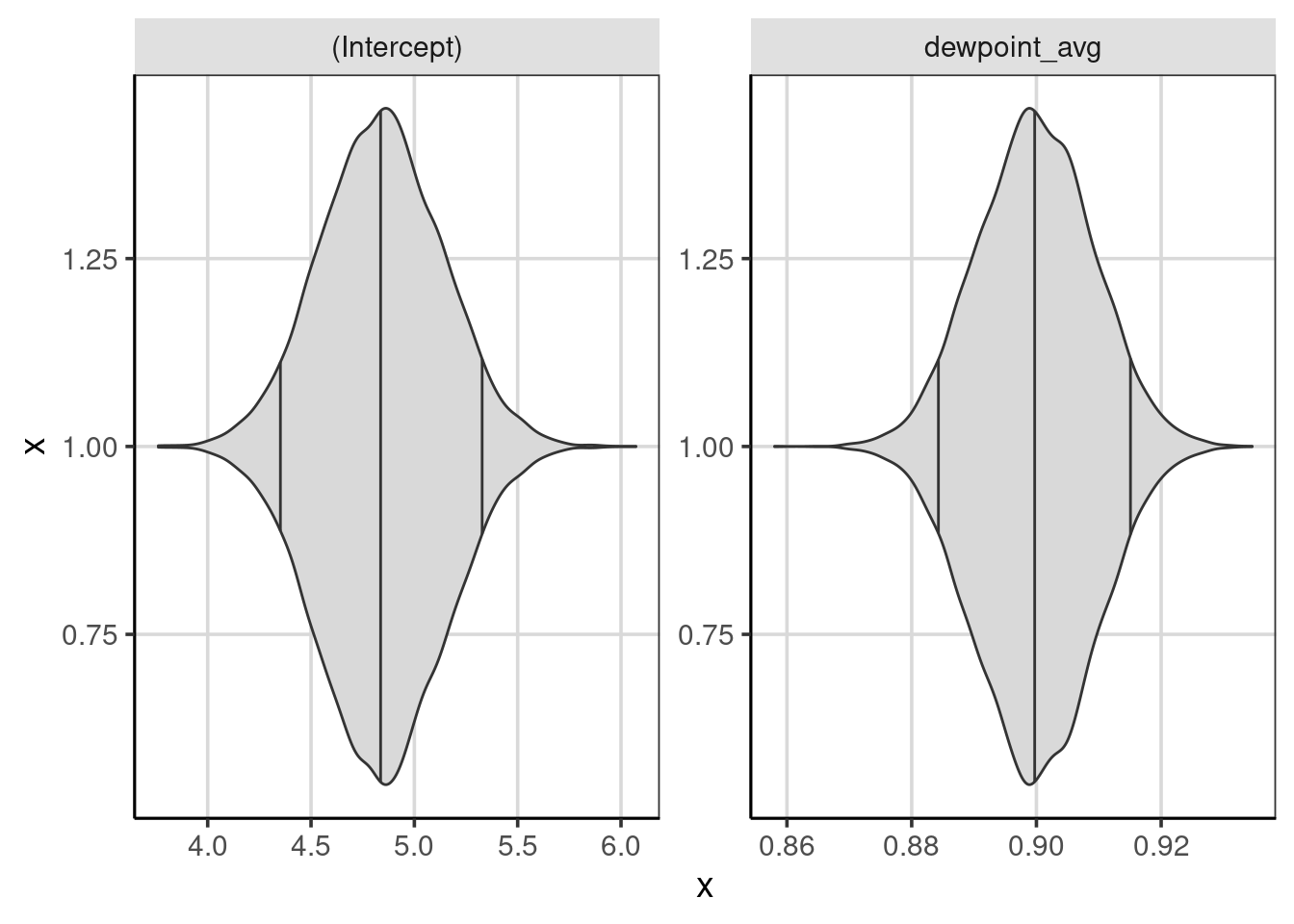# Chapter 8 Estimation, Bootstrap and Uncertainty

This section will explore how the estimates for the linear regression model are obtained. Within this section the characteristics of those estimates and the assumptions for the linear regression model will be explored.

To come …

## 8.1 Bootstrap and Uncertainty

Bootstrap and resampling methods can be used to estimate the variability in the estimated effects. This is needed as it is most common to have a subset of the entire population rather than the entire population, therefore the model estimates are approximations of the true population parameters. If another sample of data were obtained, the model estimates would be different from the previous sample. This would occur due to different individuals comprised within the sample.

The goal of a good sample is to be able to get good estimates of the population parameters of interest. This happens when the sample obtained is as representative as possible of the population, most notably this can be gathered by collected the sample using a random process or ensuring that everyone in the population has the same random chance of being selected to be in the population. This is more challenging than one would expect where practical issues can often tarnish a good sampling plan. For instance, a portion of the population of interest may be difficult to gain access to or a portion of the population may be less likely to participate. These issues could bias the sample to be dissimilar from the population. For this book, we are going to assume that a representative sample is obtained from the population, but this needs to always be explored compared whenever data is gathered.

For this chapter, the United States (US) weather data will be used again. The following code chunk loads the packages used in this chapter, sets the figure theme, and creates a few new attributes for the US weather data.

library(tidyverse)
library(ggformula)
library(mosaic)
library(broom)
library(statthink)

# Set theme for plots
theme_set(theme_statthinking(base_size = 14))

us_weather <- mutate(us_weather, snow_factor = factor(snow),
snow_numeric = ifelse(snow == 'Yes', 1, 0))

## 8.2 Estimating Error or uncertainty

To get some sense of the amount of error in the estimate of the linear slope, a bootstrap or resampling can be done to provide evidence for the likely range of slope values. This is an empirical approach to estimating uncertainty and the sampling process will be simulated many times. Upon every new data after resampling, the estimate for the linear slope will be estimated. The bootstrap/resampling will take the following general steps:

1. Resample the observed data available, with replacement
2. Fit the linear regression model, or more generally any statistic or statistical model.
3. Save the coefficient of interest.
4. Repeat steps 1 - 3 many times
5. Explore the distribution of the coefficient from the many resampled data sets.

### 8.2.1 Resample with replacement

Resampling the data with replacement, is the step in the bootstrap/resampling procedure that is needed to ensure that the estimates obtained are accurate and that there are different samples obtained throughout the process. Before moving on, this idea is worth expanding on to understand the differences and what exactly sampling with replacement means.

To do this, a simple example of fruit names will be used. An object is created with code below that has 7 fruits in it. The data also contain a made up popularity measure that shows how much individuals like each fruit. These are also printed to show that these elements each show up in the object exactly one time.

fruit <- data.frame(
names = c('apple', 'banana', 'kiwi', 'orange', 'plum', 'pear', 'kumquat'),
popularity = c(10, 25, 5, 15, 5, 8, 2)
)
fruit
##     names popularity
## 1   apple         10
## 2  banana         25
## 3    kiwi          5
## 4  orange         15
## 5    plum          5
## 6    pear          8
## 7 kumquat          2

Imagine for the setup of the resampling procedure, that these 7 fruit are placed inside a hat, box, or some container and fruit are not able to be seen. Then, to perform the resampling, someone would reach inside and select a single fruit at random. In this case, this would mean to also ignore the shape and size of the fruit as well to keep the process random. What happens next would be the difference between the two primary sampling procedures.

For sampling with replacement, the single fruit that was selected will then be placed back into the hat or box. Once the selected fruit is returned, the individual would again reach in to select another fruit at random. The fruit would then be returned to the hat or box and the individual would select another fruit at random. This process would be repeated until the same size as the original data, in this case 7 fruits, were selected randomly.

For sampling without replacement, the process is similar, except that when a fruit is selected from the box or hat it is not placed back to potentially be selected again. For example, if on the first selection with the 7 original fruit in the hat, an individual selects the kiwi, then this kiwi would not go back into the hat and could not be selected another time.

Using the fruit example above, the sample_n() function can be used to generate random samples. The default behavior of this function is to do sampling without replacement. The primary argument for the function is the data to sample from and the second argument is the number of samples to perform. In this case, the number of samples will want to match the number of elements in the original data. The replace = FALSE is an explicit way to specify that the sampling should be done without replacement.

set.seed(50)
sample_n(fruit, 7, replace = FALSE)
##     names popularity
## 1    kiwi          5
## 2  orange         15
## 3  banana         25
## 4    plum          5
## 5 kumquat          2
## 6   apple         10
## 7    pear          8

Notice that the data each show up 1 time, just like the original sample, however, the data are in a different order. This order is the order that the sampling algorithm selected each element, namely, the kiwi was selected first, then the orange, then the banana, and so on.

Sampling with replacement is now shown below and is specified by saying, replace = TRUE. The process is done twice to show what happens if

sample_n(fruit, 7, replace = TRUE)
##    names popularity
## 1 orange         15
## 2 banana         25
## 3   kiwi          5
## 4   plum          5
## 5   plum          5
## 6   kiwi          5
## 7 orange         15
sample_n(fruit, 7, replace = TRUE)
##     names popularity
## 1  banana         25
## 2 kumquat          2
## 3    plum          5
## 4  banana         25
## 5 kumquat          2
## 6  orange         15
## 7   apple         10

First, notice that each time the sampling algorithm is ran, a different set of fruits are returned. Also, notice that for each time, there are more than 1 of some of the fruit and some of the fruit do not show up. For example, in the first resampling with replacement, the orange, kiwi, and plum each show up 2 times, the banana shows up once and the remaining fruit do not show up. For the second resampling with replacement, the banana and kumquat show up 2 times and the plum, orange, and apple each show up 1 time, but the other two fruit do not show up. This is how sampling with replacement works and differs from sampling without replacement here, namely, each element could show up more than one time and that each time the sampling algorithm is performed, a different sample is obtained.

Sampling without replacement is commonly done for the selection of subjects from a population of interest. However, this procedure would not work well for the bootstrap/resampling procedure to estimate uncertainty because sampling with replacement would always produce the same sample. That is, each element of the original sample would be selected 1 time, therefore, the sample would be the same every time and the estimated statistic of interest would always be the same. This will be explored more in the next section.

### 8.2.2 Calculate statistic of interest

If these elements are saved, we could also compute the mean of the popularity column. This would represent The mean of the original sample is 10. Performing sampling without replacement, the mean will be the same as the elements within the sample will be the same as the original sample. Notice that the mean computed below with the df_stats() function is the same as the original data.

set.seed(50)

sample_n(fruit, 7, replace = FALSE) %>%
df_stats(~ popularity, mean)
##     response mean
## 1 popularity   10

However, performing sampling with replacement, these means will differ as the elements within the sample will differ. Notice below that both means are slightly larger than 10, but more importantly they differ from the original sample mean. These represent 2 of a total 8.2354310^{5} total resampled values that could be obtained. Even though this is almost a million possible resampled data samples that could be obtained, we can get a large number of these to see how much variation there can be in these statistics.

sample_n(fruit, 7, replace = TRUE) %>%
df_stats(~ popularity, mean)
##     response     mean
## 1 popularity 10.71429
sample_n(fruit, 7, replace = TRUE) %>%
df_stats(~ popularity, mean)
##     response mean
## 1 popularity   12

### 8.2.3 Replicating the resampling/bootstrap

The process of resampling without replacement and computing the statistic of interest can be combined into a single operation by creating a function that does both steps at once. This function does those steps in the second and third line of the function. You could read this function as the following, first take the data, then do the resampling of the same length (i.e., number of observation) and with replacement, and finally compute the mean of the popularity attribute.

fruit_boot <- function(...) {
fruit %>%
sample_n(nrow(fruit), replace = TRUE) %>%
df_stats(~ popularity, mean)
}

The resampling procedure can then be replicated a single time by calling this function directly. The function does not need an argument, rather the function directly calls the fruit data and the popularity attribute. Some additional programming would be needed to generalize this resampling/bootstrap function to work on other data. To show that the function is working as intended, that is sampling with replacement, the function is ran twice.

fruit_boot()
##     response     mean
## 1 popularity 14.71429
fruit_boot()
##     response     mean
## 1 popularity 10.57143

Different mean values are generated, suggesting that the sampling with replacement is working properly. Compiling the different mean values would be tedious to do by hand, fortunately, the function can be replicated many times using the computer with the map_dfr() function. This function takes the number of times to be replicated in the form of 1:replications where replications is replaced with the number of times to replicate the function.

popularity_stats <- map_dfr(1:10000, fruit_boot)

Once these are generated, the distribution of the mean fruit popularity can directly be shown using a density curve. Figure 8.1 shows this distribution which has a minimum value of 3.29 to a maximum value of ’r round(max(popularity_stats\$mean), 2) with a mean of 9.97. This indicates a range of possible values that the mean fruit popularity falls and helps to estimate the amount of uncertainty in the statistic of interest. In general, ranges that are smaller indicate less uncertainty and more accurate estimate for the statistic of interest. However, the overall range of a distribution can be misleading and by itself is not a great measure of variation. As can be seen in Figure 8.1, there is a slight positive skew in the distribution, therefore other statistics of variation are often better to truly represent the variation in the distribution.

gf_density(~ mean, data = popularity_stats) %>%
gf_labs(x = "Mean Popularity")Figure 8.1: Density curve of the mean fruit popularity based on 10,000 resampled data sets.

These statistics could be the IQR, standard deviation, or differences between other percentiles that are deemed meaningful (i.e., the difference between the 10th and 90th percentiles). In these metric, the amount of variation would be, IQR = 3.71, SD = 2.72, and difference between 10th and 90th percentiles = 7. These would be a better representation of the amount of uncertainty for this distribution and represent the range of plausible values for the distribution. For example, the SD shows that the on average most of the mean fruit popularity statistics would fall about 2.72 units away from the mean of 9.97.

### 8.2.4 Linear regression bootstrap/resampling example

An example of using the bootstrap/resampling methods with the US weather data will be explored next. In chapter 7, the minimum temperature was predicted or explained using the average daily dew point. As a reminder, here is the model again. Recall that the intercept here represents the average minimum temperature (in Fahrenheit) for an average daily dew point of 0 and that the slope term is the average increase in minimum temperature for every one unit increase in the average daily dew point.

temp_lm <- lm(drybulbtemp_min ~ dewpoint_avg, data = us_weather)
coef(temp_lm)
##  (Intercept) dewpoint_avg
##    4.8343103    0.8999469

The one element that was not explored in Chapter 7 is the amount of uncertainty, error, or variation in these estimates from the linear regression model. As shown in this chapter, the bootstrap or resampling methods can be an empirical way to try to capture the uncertainty. The steps to conduct the bootstrap or resample in this situation will be as follows.

1. Resample, with replacement, from the original US weather data
2. Fit the linear regression model with minimum temperature and average dew point
3. Save the regression coefficients
4. Repeat steps 1 - 3 many times (i.e., 10,000)
5. Visualize distribution of save regression coefficients across replications

The following function aims to do steps 1 through 3 above. First, it resamples the data with replacement of the same size as the original data. Then, using the resampled data, the linear regression model is fitted and the model coefficients are saved. The function is also ran one time to show the estimated coefficients from the resampled data. Notice how these differ from the regression model fitted above to the original data, why do these differ here?

set.seed(2021)

resample_weather <- function(...) {
weather_resample <- us_weather %>%
sample_n(nrow(us_weather), replace = TRUE)

weather_resample %>%
lm(drybulbtemp_min ~ dewpoint_avg, data = .) %>%
coef(.) %>%
broom::tidy()
}

resample_weather()
## # A tibble: 2 × 2
##   names            x
##   <chr>        <dbl>
## 1 (Intercept)  4.80
## 2 dewpoint_avg 0.904

Now that there is a function that does steps 1 - 3, these processes can now be repeated many times with the map_dfr() function. In this example, the resampling is done 10,000 times and the coefficients from each of those are saved. The distribution for the intercept and the linear slope are visualized with density curves in Figure 8.2. This figure shows that the regression slopes (right-most) figure, are distributed closely together with most of the slopes ranging from about 0.89 to 0.91. There are some that are smaller than 0.89 or larger than 0.91, but these occur less frequently than between those two values. A similar interpretation can be made for the intercept where the majority of the intercepts fall between about 4.5 and 5.25 or so.

weather_coef <- map_dfr(1:10000, resample_weather)

gf_density(~ x, data = weather_coef) %>%
gf_facet_wrap(~ names, scales = 'free') %>%
gf_labs(x = "Regression Estimates")Figure 8.2: Distribution of regression coefficients from the bootstrapped samples.

To get a more accurate sense of where notable percentiles are found, these can be computed with the df_stats() function directly. The quantile() function helps compute the percentiles of interest with the 5th, 50th (median), and 95th percentiles asked for in the code shown below. The difference between the 95th and 5th percentiles gives evidence for where the majority of the regression coefficients fall. These could be shown using violin plots for a visual depiction of the distributions with percentiles (see Figure 8.3.

weather_coef %>%
df_stats(x ~ names, quantile(c(0.05, 0.5, 0.95)))
##   response        names        5%       50%       95%
## 1        x  (Intercept) 4.3562898 4.8390742 5.3250096
## 2        x dewpoint_avg 0.8844869 0.8997507 0.9149627
gf_violin(x ~ 1, data = weather_coef, fill = 'gray85', draw_quantiles = c(0.05, 0.5, 0.95)) %>%
gf_facet_wrap(~ names, scales = 'free') %>%
gf_refine(coord_flip())`Figure 8.3: Violin plots showing the distribution of regression coefficients from the bootstrapped samples, with percentiles.

To come …